`COMPUTE! ISSUE 19 / DECEMBER 1981 / PAGE 64`

 Part Two:An Introduction To Binary Numbers Charles BrannonGreensboro, NC This is the second in a series of articles on elementary computer arithmetic. The previous article, Part One, described the binary numbering system, as used on a microcomputer. We will now delve into the use of binary numbers—adding and subtracting. We'll start with the simplest one first—addition. Besides, you have to know how to add before you can subtract. As you might have realized, binary addition should be rather simple, since you are only adding ones and zeros. The few complications involve the carry. Just to refresh you on that, here is a sample base ten addition: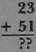To add 23 and 51, we add the numbers digit by digit, from right to left. So first we add 3 and 1 to get 4, which we place underneath the digits added. Next we add the 2 and the 5, and place a 7 under those digits to get: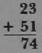The carry comes in when we add two numbers and get a result too large to fit into a single digit, as in 6 + 8. In this case we have "four, carry the one," thus: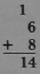Notice that the carried one drops down into the next place in the number. If we were adding 16 and 8, the carry would be added to the 1 in 16, resulting in an answer of 24. Now all of this is very elementary, but it demonstrates all the necessary actions to add in binary. Here is the "truth table" for addition in binary: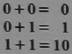The first three additions are "common sense," but the final one, 1 + 1 = 10 deserves a second look. We know that one plus one equals two, but we're working in binary, so two is expressed as one-zero, or 10 This is also equivalent to "zero, carry the one" since "10" cannot fit in a single digit. Let's run through a sample addition in binnary: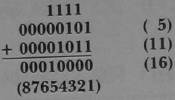1 + 1 = 0, carry the one 0 + 1 = 1, plus carry of 1 gives 0, carry the one 1 + 0 = 1, plus carry of 1 gives 0, carry the one 0 + 1 = 1, plus carry of 1 gives 0, carry the one 0 + 0 = 0, plus carry of 1 gives 1—no carry! As always, since we are working with eight-bit bytes, we fill all unused digits with zeros. This is important. As you can see, a single one can cause a whole string of carries, almost like a chain reaction. It is possible that the carry could be continued past the seventh bit (marked 8 above). Therefore, most microprocessors have a special register, called the carry bit to hold and signal this runaway bit. This bit is essential in adding multibyte numbers, which we will cover in Part Three. Let's try another addition.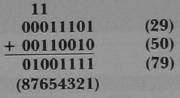This time we have an interesting effect of the carry. In step 5, we get 1 + 1=0, carry the one. In step 6, we add 1 + 1 + the carry of 1 to get 1, carry the one (1 + 1 + 1 = 11). The carry comes to rest at step 7. Incidentally, I have numbered the bits from 8 to 1 for convenience. In reality, they are numbered from 7 to 0, the exponents of the powers of two. (Bit 6=26=64). You now have the necessary information to add in binary, but in order for it to really "sink in," you will have to practice it until it becomes clear. You can make up your own exercises by randomly stringing a series of ones and zeros together to form two eight-digit numbers. Then add them in binary. To check your answer, convert the addends and the answer into decimal, which you can easily verify. When you are confident that you can add in binary, you are ready to grasp this section on subtraction. When we perform subtraction in our normal, base ten system, we are really just adding the two numbers. For example, 8—5 is equivalent to 8+ (-5). -5 is pronounced "negative five." It is assumed that you are aware of negative numbers, as it is taught as early as sixth grade, but we all can forget, right? All that is necessary is to know that, when you add a negative and a positive number, you can get the same result as subtracting the smaller number from the larger number, and giving the answer the sign of the larger number. When you add two negative numbers, the answer is the same as adding the numbers, disregarding their sign (the absolute value), and then giving the sum a negative sign (e.g. (-4) + (-3) = -7). Yet believe it or not, subtraction in binary is even easier than in decimal, as a comparison will show. First we have to know how a negative number is expressed in binary. Since a binary number is composed of ones and zeros, there is no place for the minus sign. Therefore, the highest bit, bit seven, is used to show that the number is negative. Most microcomputers use a technique called "two's complement" to convert a number into its negative equivalent. If you add numbers using two's complement, the subtraction will be performed automatically. Two's complement has two steps—forming the complement, and adding 1 to it. Numbers properly represented using two's complement are called signed binary. Let's form the signed binary equivalent of -5. Here is the binary equivalent of five: 00000101. To complement it, we turn all the zeros into ones, and all the ones into zeros to get: 11111010. Next we add 1 to it to get:Positive numbers in signed binary are expressed normally, with the restriction that they must not be greater than 127. If they were, bit seven would be "on," and the number would look as if it were negative. The number 205 in straight binary is 11001101. This is also -51 in signed binary. You can find the value of any negative number in signed binary by running it through the two's complement routine again. You'll get the positive value of the number. Similarly, if you try to make any number larger than 128 negative, it will end up positive. Therefore, in signed binary, the value in the byte must be between -128 and positive 127. Now that we have our background, let's try out our skills.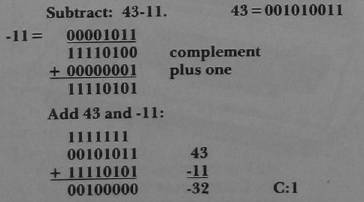Notice that the carry was swept out of the byte (C:1). C: represents the imaginary carry register. This carry should be always disregarded in two's complement subtraction. The most wonderful thing about subtraction in binary is that it is seemingly "automatic." But once again, for complete understanding, you must practice subtraction until you feel sure of your comprehension. For this purpose, exercises are once more included at the end of this article. Next time, we'll learn about multibyte numbers and even get into a wee bit of MACHINE LANGUAGE! Answers to exercises in PART ONE: 21 51 60 255 00110100 11101010 01000010 00001111 The complete chart to sixteen bits: ```32768 16384 8192 4096 2048 1024 512 256 128 64 32 16 8 4 2 1 ``` EXERCISES Add: 00101011+ 00000111 01000011+ 00011000 00111000+ 10100111 10011010+ 00111001 Convert to binary and add: 20 + 11 18 + 56 29 + 47 32 + 64 Complement only: 01010110 01100011 Form the two's complement 01111001 10111111 convert into signed binary: -14 22 -134 108 -9 Convert to binary and subtract: 56-18 99-33 58-78 -105-12 Why is -56 equal to 200? (Trick question)